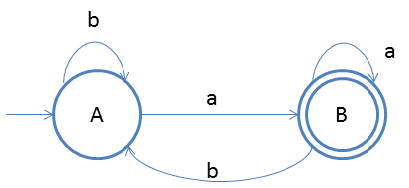DFA String Examples

Design a DFA in which set of all strings can be accepted which end with ‘a’.
Given: Input alphabet, Σ={a, b}
Language L ={aa, aba, aaabbba, abba,baba ….}

Clearly the language is infinite because there is infinite number of strings.The idea behind this approach is very simple, follow the steps below and you will understand.

1. We will first start accepting ‘b’ on start state itself and then on a we will go to final state.
2. And on final state we do not care for a’s so we have to put a self-loop.
3. On final state if ‘b’ comes then we will redirect it start state so that any string which does not end with ‘a’ should not get accepted.

Testing

1. Lets take one input string baba
2. First input is b, so from state A we will go to state A itself
3. Second input is a, so from state A we will go to state B
4. Third input is b, so from state B we will go to state A
5. Fourth input is a, so from state A we will go to state B(final state)
After end of the string we are at final state so string is accepted.